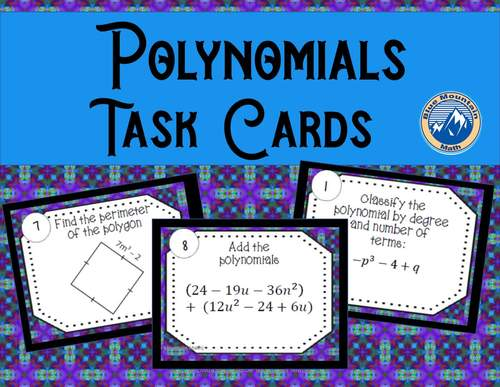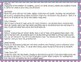# Polynomial Operations Review Task CardsSubject
Resource Type
File Type

PDF

(2 MB|14 pages)
Product Rating
Standards
• Product Description
• StandardsNEW

Students will practice identifying, adding, subtracting and multiplying polynomials in this 32 card resource. Students will also rewrite in standard form. There are many fun ways to use task cards in the classroom and I have included several of my favorite ideas. Check out the preview to see the if the questions are appropriate for your students.

The cards include the following question types:

• Identifying if an expression is a polynomial

• Classifying by number of terms and degree

• Subtracting

• Multiplying

• Writing in standard form

A recording sheet as well as a answer key is included.

Understand that rational expressions form a system analogous to the rational numbers, closed under addition, subtraction, multiplication, and division by a nonzero rational expression; add, subtract, multiply, and divide rational expressions.
Rewrite simple rational expressions in different forms; write 𝘢(𝘹)/𝘣(𝘹) in the form 𝘲(𝘹) + 𝘳(𝘹)/𝘣(𝘹), where 𝘢(𝘹), 𝘣(𝘹), 𝘲(𝘹), and 𝘳(𝘹) are polynomials with the degree of 𝘳(𝘹) less than the degree of 𝘣(𝘹), using inspection, long division, or, for the more complicated examples, a computer algebra system.
Understand that polynomials form a system analogous to the integers, namely, they are closed under the operations of addition, subtraction, and multiplication; add, subtract, and multiply polynomials.
Total Pages
14 pages
Included
Teaching Duration
50 minutes
Report this Resource to TpT
Reported resources will be reviewed by our team. Report this resource to let us know if this resource violates TpT’s content guidelines.